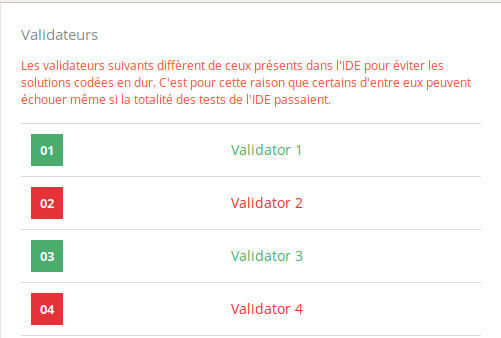# Balanced ternary computer: encode

Hell everyone. I am a noob in programming still. Now I’m stuck at puzzle “Balanced ternary computer: encode”. Actually having trouble about understanding the task.
At first we have ternary numeral system, which is based on numbers (0, 1 and 2) and after some attempts I suppose this encoding table:
{ 2: ‘1’,
1: ‘T’,
0: ‘0’, }
Some algorithm of mine works with tests 3 and 4 but still fails with tests 1 and 2.

1. have now idea about encoding input 8 to expected “10T”. I have “11” on the output
2. the same dead end about input -15 and expected “T110”. And my output is “-T10”.
Can anybody explain the encoding algorithm for me please?
Thanks anyway.

Google should bring you to suitable wiki pages.
A quick calculation, base3’s value for 4 digits are 27, 9, 3, 1
so that 10T means 19 + 1-1 = 8
and also that T110 = 27*-1 + 91 + 31 = -15

Sorry, still missing the point of task.
How in two steps of

the result contains 3 symbols of

?

And correct me, if I’m wrong: in basic ternary numerics are [0, 1, 2] and according to task it should become [T, 0, 1].
I got the first symbol for result string. Don’t want to spoil it here. And don’t know how to get the rest in correct order and quantity.

Perhaps starting with very basic examples first.
You asked why Decimal 8 equals “10T”.
Assume you know why there is a sequence 81, 27, 9, 3, 1. Here I only need [9, 3, 1].

“1” means 1 * 9
“0” means 0 * 3
“T” means T * 1 = -1 * 1
Sum the results, becomes 9-1 = 8.

Let me know if the above is still puzzling you.

Hello,

Please do you know where is the problem with my code, it pass all the tests but I don’t get 100%.

• It seems I don’t have a minor error in my code.
• It seems I have considered all the cases (I have checked the limit values).
• It seems I haven’t hardcoded.

I’ve made a test with my code, i did from -100 to 100 and I have those error with your code :

i / your code / my code

-40 / 0TTTT / TTTT
-13 / 0TTT / TTT
-4 / 0TT / TT
-1 / 0T / T
0 / 0 /
1 / 01 / 1
4 / 011 / 11
13 / 0111 / 111
40 / 01111 / 1111

I think what’s wrong is pretty obvious1 Like

Thanks for you answer but are you serious ? Test it in CodinGame, you’ll never see this error. 100% pass through the IDE but only 50% is validate. This would be impossible with this error, I think.

The IDE does not have all the cases as you’ll see. to try it i did a for loop from -100 to 100. Pass the value to your code and to mine. To fix the issue you just have to remove the first zero of your output !

It’s ok, many thanks, I just had to change "<= " by “<” in a condition and need to treat 0 separately

1 Like

Hi guys,

I am stuck in this puzzle (coding it in C)! My code passes all tests but when I submit, there is one test I am not passing (the 3rd one). Is there a way to know what value is tested for test n°3 (I don’t what to hardcode the solution but just know what values are failing)? Or does anybody want to check my code to see and tell me what is wrong with it ?

Maybe your ints are coded in only one byte.

I used the type ‘int’, so coded over 4 bytes, and I tried changing it to ‘long’ did not solve it…

Hello everyone.

I come here to share one of my problem. All tests pass in the IDE, but not in the validator.

Here is a screen of my methodology to solve this exercise. For a given number, i calculate its index in a range between sum_of_power(3i) and sum_of_power(3(i+1)). The index is equal to the number minus the sum of the power of 3 to the index i.

Once I have the index, for each power of 3 I calculate : (index%3i) / 3i.
I know that if this calculus gives me a number equals to 0 or greather than 2/3, then for this unit it is a “T” if n < 0 or a “1” if n > 0.
If this calculus give me a number inferior or equals to 1/3, then for this unit it is a “1” if n < 0 or a “T” if n > 0.
Else, this unit is equal to 0.
This is all summed up on my screen above.

I obtain everything right with this algorithm :

Even with large number:

But still I can’t figure out why the validators don’t pass.
PS : I code in Python

Thanks for your helpAre there negative numbers in this puzzle?

Yep there are, and my code works in the IDE for negative numbers.

My work on the algorithm with positive and negative numbers :

Test in the IDE with a negative number : -255

Validators that don’t pass :When I try my code in my linux terminal, it works fine. Even for large negative numbers.

Can you send me your script in private?
Anyway, are you sure that −5=TTT?

1 Like

Oups, I copied the wrong columns when I changed of sheets:

That’s better

Your script should pass the validators.Just a little message to say that I found the error in my code and now this puzzle is solved! Thanks for your help nicola1 :D.

2 Likes